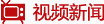栏目ID=318的表不存在(操作类型=2)
栏目ID=318的表不存在(操作类型=0)
栏目ID=336的表不存在(操作类型=2)
栏目ID=336的表不存在(操作类型=0)

# 四川北新大弘集团总裁刘邦成 赴武胜雪中送

11月28日，四川北新大弘集团总裁刘邦成不辞辛劳分别赶赴武胜县中心镇、真静乡、北坪乡开展爱心捐赠活动。武胜县政...[详细]

# 我国首批舰载战斗机飞行员通过航母资格认

据新华社电 我国第一艘航空母舰辽宁舰顺利完成今年首次预定科研试验和训练课目，昨天返航停靠青岛某军港。在这次为...[详细]

# 新疆奖励上交涉恐涉暴物品：炸药每公斤10

天山网讯 为配合集中收缴危爆物品、管制刀具、涉恐涉暴宣传品行动顺利进行，广泛调动群众参与到专项行动中来，新疆...[详细]

#### 栏目ID=12的表不存在(操作类型=2)

栏目ID=12的表不存在(操作类型=2)

#### 栏目ID=21的表不存在(操作类型=2)

栏目ID=21的表不存在(操作类型=2)

#### 栏目ID=8的表不存在(操作类型=2)

栏目ID=8的表不存在(操作类型=0)

栏目ID=12的表不存在(操作类型=1)
栏目ID=21的表不存在(操作类型=1)栏目ID=323的表不存在(操作类型=0)

栏目ID=264的表不存在(操作类型=2)

栏目ID=54的表不存在(操作类型=2)
栏目ID=54的表不存在(操作类型=2)

栏目ID=54的表不存在(操作类型=0)

栏目ID=98的表不存在(操作类型=2)

栏目ID=214的表不存在(操作类型=2)

栏目ID=212的表不存在(操作类型=2)

栏目ID=30的表不存在(操作类型=2)

栏目ID=185的表不存在(操作类型=2)

栏目ID=133的表不存在(操作类型=2)